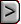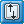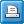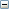﻿ STOB - Stream Observation Package
Online Guide to MODFLOW

STOB - Stream Observation PackageSTOB - Stream Observation PackageSTOB - Stream Observation PackageAbbreviation in Name file

STOB

Purpose

The Stream Observation input file is to specify observations of flow through Stream boundaries for use in the Observation process.

Documentation

 • str6.pdf
 • release.txt
 • OBS.pdf

Related Packages

 • Stream Package
 • Observation Process input files

Supported in

 • MODFLOW-2000
 • MODFLOW-2005
 • MODFLOW-LGR
 • MODFLOW-CFP
 • MODFLOW-NWT

Other Notes

The Stream (STR) package must be used in models in which the Stream Observation input file is used.

Input Instructions

Input for the Observation Process STR Package is read from a file that is specified with "STOB" as the file type listed in the name file. Free format is used throughout.

Data Set 0

[#Text]

Item 0 is optional and can include as many lines as desired. Each line needs to begin with the “#” character in the first column.Text
 Text—is a character string (maximum of 79 characters) that starts in column 2. Any characters can be included in Text. The “#” character needs to be in column 1. Text is printed when the file is read and provides an opportunity for the user to include information about the model both in the input file and the associated output file.

Data Set 1

 MODFLOW-2000: NQST NQCST NQTST (free format) MODFLOW-2005: NQST NQCST NQTST IUSTOBSV [NOPRINT] (free format) MODFLOW-CFP, MODFLOW-NWT, MODFLOW-OWHM, and MODFLOW-LGR: NQST NQCST NQTST IUSTOBSV (free format)Explanation of variablesNQST
 NQST—is the number of cell groups for which stream observations are listed. A group consists of the cells needed to represent one flow measurement (eq. 5).NQCST
 NQCST—is greater than or equal to the total number of cells in all cell groups. NQCST must be greater than or equal to the sum of all of the cells listed in all cell groups; that is, NQCST needs to exceed the sum of the absolute values of all of the NQCLST variables in the repetitions of item 3.NQTST
 NQTST—is the total number of stream observations for all cell groups. NQTST must equal the sum of all NQOBST, which are specified in repetitions of item 3 in the input file.IUSTOBSV
 IUSTOBSV (MODFLOW-2005 and MODFLOW-LGR)—File unit for saving observation data in a file. Specify 0 for no observation output file. The file for this unit must be included as type “DATA” in the Name File.NOPRINT
 NOPRINT—is an option keyword that turns off printing of input and output data in the Listing File.

Data Set 2

 MODFLOW-2000: TOMULTST  EVFST IOWTQST (free format) MODFLOW-2005, MODFLOW-CFP, MODFLOW-NWT, MODFLOW-OWHM, and MODFLOW-LGR: TOMULTST (free format)Explanation of variablesTOMULTST
 TOMULTST—is the time-offset multiplier for stream observations [-- or T/T]. The product of TOMULTST and TOFFSET must produce a time value in units consistent with other model input. TOMULTST can be dimensionless or can be used to convert the units of TOFFSET to the time unit used in the simulation.EVFST
 EVFST— is the error variance multiplier for observations represented using the Stream Package, and is used to calculate the weights as described below in the explanation of STATISTIC. EVFST makes it easy to change the weights uniformly for all flow observations represented using the Stream Package.IOWTQST
 IOWTQST— is a flag that indicates that the variance-covariance matrix on STR-Package flow observations is to be read into array WTQ of item 7. If IOWTQST equals zero, weights are calculated using STATISTIC of item 4; if it is greater than zero, items 6 and 7 are read and used to calculate the weights.

Read items 3, 4, and 5 for each of NQST groups of cells for which stream observations are to be specified.

Data Set 3

NQOBST NQCLST (free format)Explanation of variablesNQOBST
 NQOBST—is the number of times at which flows are observed for the group of cells.NQCLST
 NQCLST—is a flag, and the absolute value of NQCLST is the number of cells in the group. If NQCLST is less than zero, FACTOR = 1.0 for all cells in the group.

Data Set 4

 MODFLOW-2000: OBSNAM IREFSP TOFFSET HOBS STATISTIC STAT-FLAG PLOT-SYMBOL MODFLOW-2005, MODFLOW-CFP, MODFLOW-NWT, MODFLOW-OWHM, and MODFLOW-LGR: OBSNAM IREFSP TOFFSET FLWOBS

Read item 4 for each of NQOBST observation times for this group of cells. STATISTIC and STAT-FLAG are ignored if IOWTQST is greater than zero.Explanation of variablesOBSNAM
 OBSNAM—is a string of 1 to 12 nonblank characters used to identify the observation.  The current version of MODFLOW-2000 (v 1.17.02) accepts duplicate observation names, but if duplicate names are found, a warning concerning the duplication is written to the Global file.IREFSP
 IREFSP—is the reference stress period to which observation times are referenced. The reference point is the beginning of the stress period.TOFFSET
 TOFFSET—is the time offset of the observation, from the beginning of stress period IREFSP [T]. TOFFSET must be in units such that the product of TOMULTST and TOFFSET is in time units consistent with other model input. TOFFSET and TOMULTST from the STOB file and values of PERLEN, NSTP, and TSMULT from the DIS file are used to determine the stress period, time step, and time during the time step for the observation. To specify that an observation is for a steady-state model solution, specify IREFSP as the stress-period number of the steady-state stress period, and specify TOFFSET such that TOMULTST ×TOFFSET is less than or equal to PERLEN for the stress period; if PERLEN is zero, set TOFFSET to zero. If the observation falls within a time step, the simulated equivalent is calculated by linearly interpolating between values for the beginning and end of the time step. If the first stress period is transient and the observation falls within the first time step, the simulated equivalent from the end of the time step is used because no flow from the beginning of the time step is available for interpolation.FLWOBS
 FLWOBS (MODFLOW-2005 and MODFLOW-LGR)—is the observed seepage from the stream into the aquifer (positive) or the discharge from the aquifer into the stream (negative) [L3/T].HOBS
 HOBS (MODFLOW-2000)—is the observed stream-boundary gain (if HOBS is negative) or loss (if HOBS is positive) [L3/T]. The terms ‘gain’ and ‘loss’ are from the perspective of the surface-water body, so that gains occur when water leaves the groundwater system, and losses occur when water flows into the groundwater system.STATISTIC
 STATISTIC (MODFLOW-2000)—is the value from which the weight for the observation is calculated, as determined using STAT-FLAG. STATISTIC is ignored if IOWTQST is greater than zero, in which case WTQ of item 7 is used to define the weighting.STAT-FLAG

STAT-FLAG (MODFLOW-2000)—is a flag identifying what STATISTIC is and how the weight is calculated. STAT-FLAG is ignored if IOWTQST is greater than zero.

 • STAT-FLAG = 0, STATISTIC is a scaled variance [(L3/T)2], weight = 1/(STATISTIC × EVFST)
 • STAT-FLAG = 1, STATISTIC is a scaled standard deviation [L3/T], weight = 1/(STATISTIC2 × EVFST)
 • STAT-FLAG = 2, STATISTIC is a scaled coefficient of variation [--], weight = 1/[(STATISTIC × HOBS)2 × EVFST]PLOT-SYMBOL
 PLOT-SYMBOL (MODFLOW-2000)—is an integer that will be written to output files intended for graphical analysis to allow control of the symbols used when plotting data.

Data Set 5

SEGMENT REACH FACTOR (free format)

Read item 5 for each cell in this group; the number of cells is equal to the absolute value of NQCLST read in item 3.Explanation of variablesSEGMENT
 SEGMENT—is the segment number of a stream cell included in the cell group.REACH
 REACH—is the reach number of a stream cell included in the cell group.FACTOR
 FACTOR—is the portion of the simulated gain or loss in the cell that is included in the total simulated gain or loss for an observation (fn of eq. 5).

Read items 6 and 7 if IOWTQST is greater than 0. Items 6 and 7 are not available in MODFLOW-2005 and MODFLOW-LGR.

Data Set 6

FMTIN IPRN (free format)Explanation of variablesFMTIN
 FMTIN—is the Fortran format to be used in reading each line of the variance-covariance matrix used to calculate the weighting. The format needs to be enclosed in parentheses and needs to accommodate real numbers.IPRN

IPRN—is a flag identifying the format in which the variance-covariance matrix is printed. If IPRN is less than zero, the matrix is not printed. Permissible values of IPRN and corresponding formats are:

 Output requires more than 80 columns Output requires 80 columns or less IPRN FORMAT IPRN FORMAT 1 10G12.3 6 5G12.3 2 10G12.4 7 5G12.4 3 9G12.5 8 5G12.5 4 8G13.6 9 4G13.6 5 8G14.7 10 4G14.7

Data Set 7

WTQ(1,1), WTQ(1,2), WTQ(1,3), ... , WTQ(1,NQTST) (format: FMTIN)

WTQ(2,1), WTQ(2,2), WTQ(2,3), ... , WTQ(2,NQTST)

...

WTQ(NQTST,1), WTQ(NQTST,2), WTQ(NQTST,3), ... , WTQ(NQTST,NQTST)WTQ
 WTQ—is an NQTST by NQTST array containing the variance-covariance matrix on STR-Package flow observations [(L3/T)2 ]. For elements WTQ(I,J), if I ≠J, WTQ(I,J) is the covariance between observations I and J; if I = J, WTQ(I,J) is the variance of observation I. Note that the variance-covariance matrix is symmetric, but the entire matrix (upper and lower parts) must be entered.